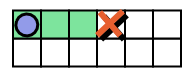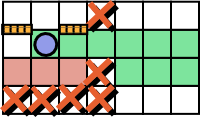## Wednesday, May 29, 2013

### SRM 580, WallGameDiv2, editorial preview

Here is a preview of 579's editorial. Begin with division 2 hard. The editorial page contains this explanation and once the rest of the editorial is finished it might contain an updated/fixed version. This page is just the preview.

I intended to write this one even since Saturday . But I had difficulty coming up with an explanation that doesn't just jump to "calculate the worst path, duh!".

## WallGameDiv2

Link to the problem statement. There is a board with digits (as costs) in some cells and letter x in the remaining cells. In the first turn, Eel can build some walls (possibly none) between any two vertically-adjacent cells. In the second turn, Rabbit places a token in the top-left (0,0) cell, then Rabbit gets to move the cell down, left or right to any existent cell that does not contain a X, the cost to do the move is equal to the digit written in the cell. The game finishes when Rabbit takes the token to the bottom row. Eel cannot place the walls in such a way that it is not possible for Rabbit to find an exit. Rabbit wants to spend as little as possible and Eel wants Rabbit to spend as much as possible. Return the total cost Rabbit pays if both Rabbit and Eel play optimally.

## Eel's control of the game

Let us play from Eel's perspective. Rabbit starts at top left cell and may be able to move to many cells in the top row until a blocked cell or the border of the board:In an example with three reachable cells in the top row, the Rabbit can move to any of them and them move to the cell bellow it. Ad Eel we can place walls to vertically sperate cells from each other. With the help of walls we can actually force the Rabbit to move to a specific one of them:With the help of walls we can assume that the Rabbit will have to move the token to the position in the second row that we wanted. There are once again three locations in the lower row that are reachable. One of the other location is blocked with an 'x'. The remaining locations (in red) are not valid, because if we made the Rabbit move there, the Rabbit would be unable to reach the bottom row (The paths from each of the squares in that area to the bottom row are blocked). Although we have many valid locations where we can force the Rabbit to move, we should place walls in a way that maximize the cost. We must leave at least one of them open for the Rabbit, though:

Then we face other three choices where to put the wall gap. The image to the right shows the whole path the Rabbit took to reach the bottom row.

The path the Rabbit takes at the end is a simple path, the Rabbit never visits the same square twice. There is no reason to, because the Rabbit already knows the positions of all the walls and can take a direct route. The simple path will finish at any of the cells in the bottom row.

Although the previous logic to consider sub-problems with the starting cell in each row in mind allows a dynamic programming solution. If you instead notice that Eel can force Rabbit to take any simple path to the bottom row that Eel prefers we can have a simpler one. For example, consider the following simple path:It is possible to decide walls that force Rabbit to take any valid simple path we want. Eel wants Rabbit to spend the maximum cost possible. The path Eel forces Rabbit to take should be the one that has the largest possible sum of cell values, the worst simple path (It must be valid though, it must finish at one of the cells in the bottom row.).

## Worst valid path from (0,0) to the bottom

A path is a sequence of cells. A simple path is a sequence of cells that does not visit the same cell twice. We can try a backtracking logic: The fist cell in the path is (0,0). When the current cell is (x,y), there are up to three choices for the next cell in the path:

• (x,y+1) : Move down
• (x - 1,y) : Move left.
• (x + 1,y) : Move right.

In many cases some of these moves are invalid. The new cell could have a 'x' and be blocked. It is also possible that no valid path is possible if you move to that cell. Or maybe the cell does not exist (you are at one of the borders and can only move in one horizontal direction). Or maybe the cell was already visited by the path, which would not be good because the path must be simple. Because we can't move up, the only way to visit a cell that was already visited is to move backwards - Move left after a move to the right or vice versa. Thanks to this we only need to remember three variables: (x,y) the current cell and d, the previous direction. For example d is 1 if the previous move was to the left, 2 if the previous move was right and 0 otherwise. The result is a recurrence relation f(x,y,d) that returns the worst path starting at (x, y) such that the new direction is consistent with d:

• f(x,h - 1,d) = (cost(x, h-1)) (If h is the height of the board, then all cells at row (h - 1) are in the bottom. The path has finished.
• f(x, y, d) = -INF (A very small negative number) in case there are no valid paths starting at (x,y) such that the next direction is consistent with d. If (x, y) is "x" assume also that the result is -INF. We use -INF to mark this state as invalid, because the latter steps maximize the result, so any valid result will be greater than the invalid result.
• f(x, y, 0) = cost(x, y) + (The worst path out of f(x, y+1, 0), f(x-1, y, 1), f(x+1, y, 2) ). (E.g: If we decide to move right, the new value of d is 2, and the next starting cell is (x+1, y).
• f(x, y, 1) = cost(x, y) + (The worst path out of f(x, y+1, 0) or f(x-1, y, 1) ). (The previous move was to the left, it is not valid to move right).
• f(x, y, 2) = cost(x, y) + (The worst path out of f(x, y+1, 0) or f(x+1, y, 2) ). (The previous move was to the right, it is not valid to move left).

This recurrence relation is a cyclic and has a limit number of state combinations O(w*h) (There are at most three values of d for each cell). We can use memoization to implement it in polynomial time.

vector<string> costs;int w, h;int dp;int worstPath(int y, int x, int d){    const int INF = 1000000000;    int res = dp[y][x][d];    if (res == -1) {        int c = costs[y][x]-'0';        if ( costs[y][x] == 'x') {            // Invalid            res = -INF;        } else if (y == h-1) {            // Base case, the bottom is the end of the road:            res = c;        } else {            // Move down:            res = c + worstPath(y+1, x, 0);            // Move left (as long as the previous move was not right)            if ( (d != 2) && (x > 0) ) {                res = std::max(res, c + worstPath(y, x-1, 1) );            }            // Move right (as long as the previous move was not left)            if ( (d != 1) && (x < w-1) ) {                res = std::max(res, c + worstPath(y, x+1, 2) );            }        }        // memoize the result:        dp[y][x][d] = res;    }    return res;}int play(vector <string> costs){    //Save some variables for use by the worstPath function:    this->costs = costs;    w = costs.size();    h = costs.size();    // initialize the whole dp[][][] array with -1:    memset(dp, -1, sizeof(dp));    // Solution: x=0, y=0, d=0    return worstPath(0,0, 0);}

#### 1 comment :Skakar said...

Grear worj